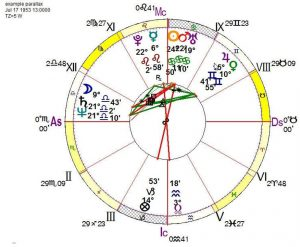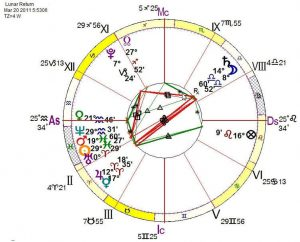## To Parallax or not to Parallax – Something to think about

### By Alphee Lavoie

A normal way for astrologers to calculate a chart is to do so as if all of the planetary positions show us being born in the center of the earth. However, none of us are not born in the center of the earth. If you were to calculate all of the planets at your exact birth locality, which is known as parallax, this is technically the correct way of calculating the planetary positions. The closer a point is to the earth the more that the position will change using parallax. Chart A was calculated without parallax while chart B was calculated with parallax. Notice that the two charts have the exact same house cusps. The Sun’s position only changed by a few seconds as did Mercury and Venus. (seconds are not shown on this chart but they did change). But the major difference using parallax is the position of the Moon.

Chart A shows the Moon’s position to be 8° 52 min. of Libra. On the other hand chart B calculated with parallax shows the Moon’s position as 9° 43 min. of Libra. That’s a difference of 51 minutes which equals almost a full degree. If you were using solar arcs as a prediction technique it would make the Moon’s aspect to any points in the chart off by almost a year.

# CHART A# CHART BNow let’s take a look at the two different lunar returns for March 20, 2011. Chart C is calculated without using parallax in the natal chart. The T-square involves the second, eighth and eleventh houses. It seems like the stress for the month would be more around money. The planets in the Tsquare are in fixed houses indicating a more material, physical theme.

Chart D was calculated using parallax in the natal chart. Note that the planets in the T-square fall in angular houses which deal with more personal issues, relationships and career. It’s a completely different accent than the lunar return above without the parallax calculation.

I don’t know the exact percentage but using parallax will not change all charts in a radical way, however, in the ones that it does change the difference is substantial.

For example the difference in the Moon in my natal chart using parallax is 6 minutes and in the lunar return that would change the lunar return Ascendant by 1 degree. In my wife chart the difference between the two Moons is 30 minutes. That produce a big difference in her Lunar returns.

CHART CCHART D